# Bihar Board 12th Accountancy Important Questions Short Answer Type Part 5 in English

BSEB Bihar Board 12th Accountancy Important Questions Short Answer Type Part 5 are the best resource for students which helps in revision.

## Bihar Board 12th Accountancy Important Questions Short Answer Type Part 5 in English

Question 1.
From the following information., prepare a Comparative Income Statement: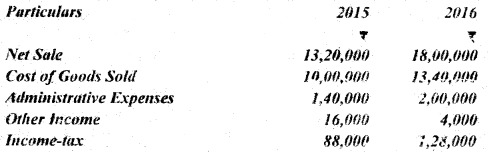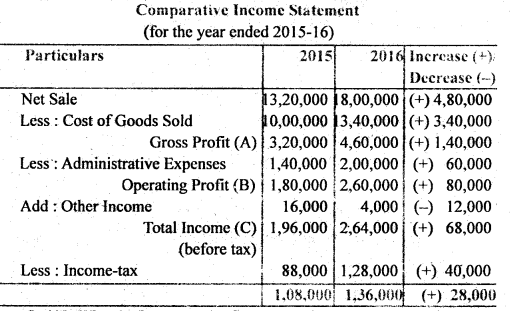Question 2.
What is Common-size Statements?
Meaning : Common-size statement is a statement expressing all items of a financial statement as a percentage of some measure of size of the enterprise. Thus, Common-size financial statement shows the percentage of each item to the total in each period. If the Balance Sheet and Income Statement items are shown in analytical percentage i.e., percentages or ratios to the total of appropriate items (total assets, total liabilities and total net sales) a common base for comparison is provided. The statements in this form are called Common-size Statements. Such statements are useful in studying the comparative financial position of two or more businesses. In Common-size Statements vertical analysis is required.

Common-size statements are often called ‘component percentage’ or ‘100 percent’ statements because each statement is reduced to the total of 100 and each individual item is stated as a percentage of the total of 100.
Common Size% = $$\frac{\text { Each Item of Revenue }}{\text { Net Sales }} \times 100$$

Question 3.
What are the limitations of Common-size Statements?
Following are the main limitations of common-size statements :

1. Difference between Different Activities : The use of this method is not possible when the various activities of a concern differ from each other.
2. Difference in Accounting Methods : If the accounting methods followed by two businesses are different, this method of analysis can not be used.
3. Difference in Heads of Account: If there is a difference between the various heads of accounts, i.e., different expenses are treated and written or collected, this method may give misleading conclusions.

Question 4.
Prepare common-size income statement of AB Ltd. with the help of the following information :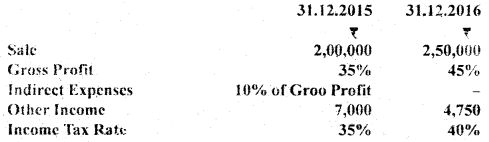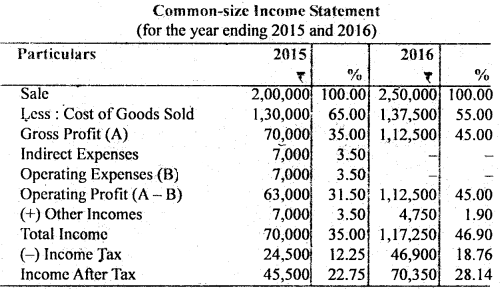Question 5.
Ramu Ltd. has a current ratio of 3 : 1. If its stock is ₹ 30,000 and total current liabilities are ₹ 60,000, find out its stock.
Liquid Ratio = $$\frac{\text { Liquid Assets }}{\text { Current Liabilities }}$$
Given : Current Ratio = 3 : 1
Current Liabilities = ₹ 60,000
∴ Current Assets 60,000 × 3 = ₹ 1,80,000
Liquid Assets = Current Assets – Stock
= ₹ 1,80,000 – 30,000
= ₹ 1,50,000
Quick Ratio/Liquid Ratio = $$\frac{1,50,000}{60,000}$$ = $$\frac{15}{6}$$ or 2.5 : 1

Question 6.
AB Ltd. has a current ratio of 4.5 : 1 and a quick ratio of 3 : 1. If its stocks is ₹ 36,000, find out its Current Assets and Current Liabilities.
Let C.L. = x
∴ C.A. = 4.5x
L.A. = 3x
Stock = CA – L.A.
36,000 = 4.5x – 3x
36,000 = 1.5x
∴ x = $$\frac{36,000}{1.5}$$ x = 24,000
∴ C.L. = ₹ 24,000
C.A. = 4.5x = 4.5 × ₹ 24,000 = ₹ 1,08,000
C.A. = ₹ 1,08,000

Question 7.
What is Profitability Ratio? Explain.
Profitability Ratio : Profitability refers to the ability of a business to earn profit. It shows the efficiency of the business. These ratios measure the profit earning capacity of the company. Profitability has direct link with sales. This is why, we calculate these ratios on the basis of sales. Return on investments and capital is calculated on the basis of capital employed. Generally profitability ratio is calculated in percentage (%).

Generally, three types of profitability ratios are calculated :

1. Profitability Ratios based on Sales.
2. Profitability Ratios based on Investments or Capital Employed.
3. Profitability Ratios based on Earning on Shares.

Some important profitability ratios are as under :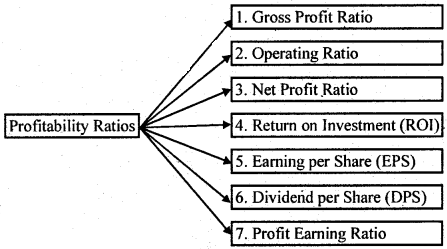Question 8.
Explain any three limitations of the Ratio Analysis.
The limitations of Ratio Analysis are as follows :

1. False Results : Rati0s are based upon the financial statements. In case, financial statements are incorrect or the data upon which ratios are based is incorrect, ratios calculated will also be false and defective.
2. Limited Comparability : The ratio of the one firm can not always be compared with the performance of other firm, if uniform accounting policies are not adopted by them.
3. Lack of Standard Universally accepted Terminology : The ratio can be comparable only when uniform terminology is adopted by both the firms.

Question 9.
What does Debt-Equity Ratio indicate?
Debt-Equity Ratio : Debt-equity Ratios shows a relationship between long-term debts and shareholders’ funds. In other words, this ratio indicates the relationship between outsiders’ funds (i.e., lenders’ contribution) and shareholders’ funds (i.e., owner’s contribution). It is also known as External-Internal Equity Ratio. This ratio is calculated to measure the relative claims of outsiders and the shareholders against the firm’s assets.

Computation : It is calculated on the basis of the following formula :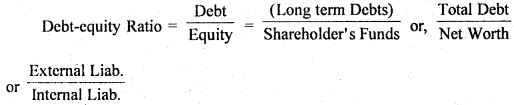Shareholder’s Funds or Net Worth or Equity Debt or Long term Debt = (Equity Share Capital + Preference Share Capital + Capital Reserve + Revenue Reserve + Reserve for Contingencies + Retained Earnings + Sinking Funds) – Fictitious Assets such as accumulated losses and deferred expenses
= Debenture + Mortgage Loans + Bank Loan + Public Deposits and Other Long-term Loan

Question 10.
From the following particulars, calculate the Inventory Ratio for each year :(i) Cost of Goods Sold = Opening Stock + Purchase – Closing Stock
For 2009-10 = ₹ 40,000+ 2,55,000 – 65,000 = ₹ 2,30,000
For 2010-11 = ₹ 65,000 + 3,40,000 – 50,000 = ₹ 3,55,000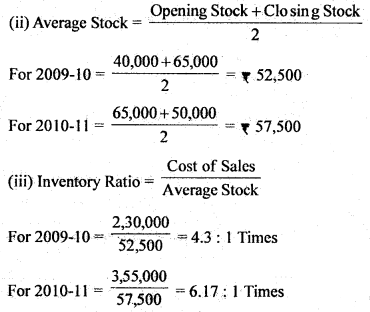Question 11.
Calculate the Gross Profit Ratio from the following :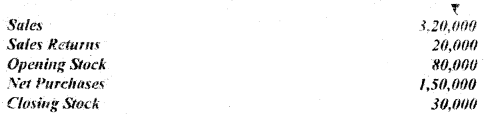Cost of Goods Sold = Opening Stock + Net Purchases – Closing Stock
= ₹ 80,000 + 1,50,000 – 30,000
= ₹ 2,00,000
Net Sales = ₹ 3,20,000 – 20,000= ₹ 3,00,000
Gross Profit = ₹ 3,00,000 – 2,00,000 = ₹ 1,00,000
Gross Profit Ratio = $$\frac{1,00,000}{3,00,000}$$ × 100 = $$33 \frac{1}{3} \%$$

Question 12.
What do you mean by Cash How Statement ? Explain.
Meaning of Cash Flow Statement : A statement of change in financial position on cash basis is commonly known as the cash flow statement. It shows the changes in cash position from one accounting period to another. In other words, cash flow statement summarises the causes of changes in cash and cash equivalents position between dates of two Balance Sheets. It indicates the source and uses of cash. This statement attempts to analyse the transactions of the firm in terms of cash.

Thus, a Cash Flow Statement can be defined as a statement which summarises sources of cash inflows and uses of cash outflows of the firm during a particular period of time, say, a month or a year.

Question 13.
How is Cash flow from Operating Activities calculated?
Calculation of Cash Flow from Operating Activities: Almost all the companies prepare its Income Statement on accrual basis of accounting under which revenues are recorded when earned and expenses are recorded when incurred. Because of this, net profit, as shown by Income Statement will not be equal to cash generated by operating activities. Hence, there is aneed to calculate cash flows from operating activities. AS-3 (Revised) suggests two methods of reporting cash flows from operating activities :

1. Direct Method, and
2. Indirect Method.

1. Direct Method : Under direct method major classes of gross cash receipts and gross cash payments are considered. Under this method, cash receipts from operating, activities, i.e., cash received from the sale of goods and rendering services, cash collected from customers and cash received from trading commission and royalties and cash payments for operating activities such as payment to creditors for goods and services, employees for their services etc. are disclosed. The difference between cash receipts and cash payments is the net cash flow from operating activities. A cash flow statement based on direct method is a Profit & Loss Account on cash basis.

2. Indirect Method : Under this method, net profit or loss is adjusted for the effects of transactions of non-cash nature and non-operating nature and changes in current assets and liabilities.

Question 14.
What is Cash Flow from Investing Activities?
Cash Flow from Investing Activities : Cash flow from investing activities are the cash flows from the transactions involving purchase and sale of resources, i.e. long-term productive assets intended to generate future income and cash flows.

Examples of Cash Flow from Investing Activities : AS-3(Revised) :

• Cash payments to acquire fixed assets including intangible assets such as goodwill, patents and copyrights. It also includes payment made to construct fixed assets.
• Cash receipts from disposal of fixed assets including intangibles.
• Payment to acquire shares or debentures, investments.
• Cash receipts from sale of shares, debentures etc. of other companies.

Question 15.
What are the objectives of Cash Flow Statement?
The main objectives of preparing Cash Flow Statement are as follows:

• To throw light on specific sources of Cash Flow.
• To ascertain the specific uses.
• To ascertain the net change in Cash and Cash Equivalents.
• To disclose changes in cash position.
• To determine cash requirement.
• For efficient cash management.
• To judge liquidity position.
• To help in short-term planning.
• To analyse financial position.
• To help in dividend decision.

Question 16.
From the following particulars, compute cash in operation: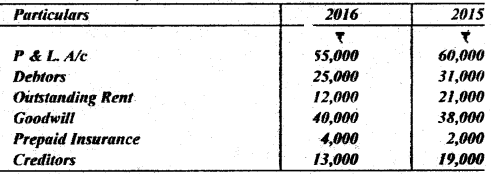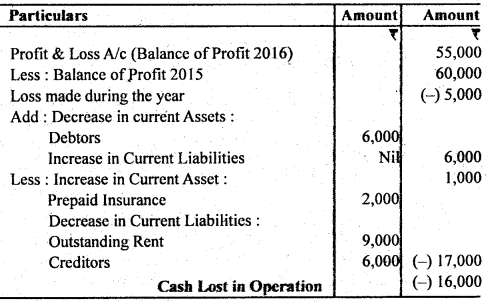Question 17.
Calculate cash flow from the following financing activities :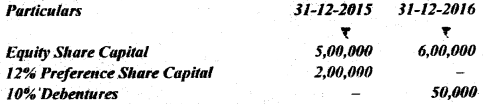Additional Information : (i) Equity shares were issued at a premium of 20%. (ii) 12% preference shares were redeemed at a premium of 10%. (iii) 10% Debentures were issued at a discount of 1%. (iv) Interim dividend paid of equity shares ₹ 90,000.
Calculation of Cash Row From Financing Activities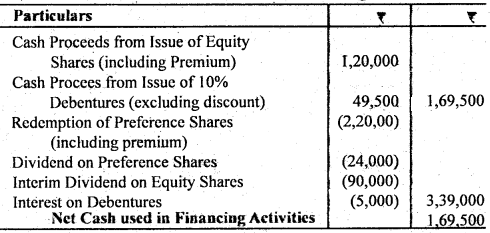Assumptions :

• Preference shares are redeemed at year end.
• Debentures are issued at beginning of year.

Question 18.
The following is the position of Current Assets and Current Liabilities of Vijay Ltd. :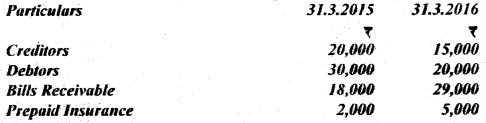The company incurred a loss of ₹ 6,000 during the year. Calculate Cash Flow from Operating Activities.
Calculation of Cash Row from Operating Activities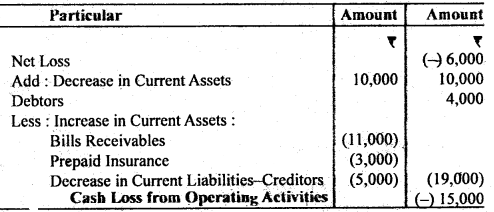Question 19.
From the following particulars, calculate Cash How from Investing Activities: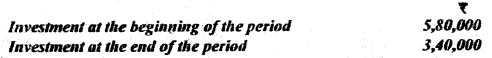During the year the company had sold 50% of its investment held in the beginning of the period at a profit of ₹ 90,000.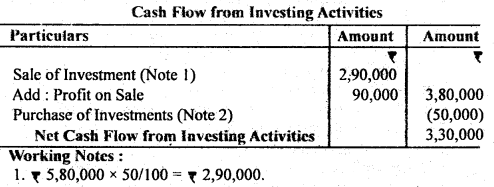2. Investment Account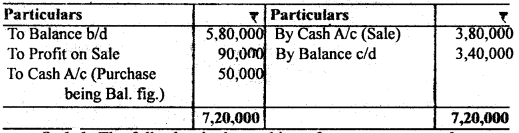Question 20.
The following is the position of current assets and current liabilities of X Ltd.: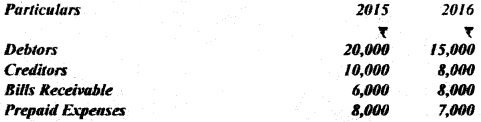The company has incurred a loss of ₹ 50,000 during the year. Calculate cash from operations.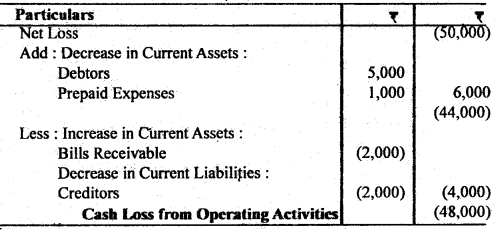Question 21.
State the features of income and expenditure A/c.
Features of income & expenditure A/c :

• It is a normal account.
• It is prepared from the receipts and payments account and other relevent information (or additional information)
• All revenue expenses related to current year are recorded on the debit side of the income & expenditure A/c.
• All revenue incomes related to current year are shown on the credit side of income & expenditure A/c.
• Items of capital nature are not shown in this account,
• Like P/L A/c it also includes the non-cash items, such as depreciation, provision for bad and doubtful debts etc.
• It shows income & expenditure of current year only on accrual basis.
• The balance at the end of the year represents surplus or deficit.

Question 22.
State the uses of Cash Flow Statement?
Along with balance sheet and income statement, the investment of cash flows would assits the users of financial statements the following way :

• To analyse the reasons for change in cash balance of the enterprise.
• To judge the enterprise ability to pay its debts, to pay dividends to its shareholders and to pay interest on loans.
• To assess the enterprise’s need to borrow cash.
• To find out the reasons for difference between the enterprise net income, cash payment and cash receipts.
• To analyse reasons for change in enterprise’s financial position including investing and financial activities during a period of time.

Question 23.
Explain three factors affecting goodwill.
Three factors effecting goodwill are as follows :

• Efficiency of management: If the business is managed by capable and competent persons, then the value of goodwill will be high.
• Nature of goods : If the business of a firm is of the nature where the goods dealt in are in high demand although not in short supply. The profits will be higher. It results is increase in the value of goodwill.
• Location : If the business is located at a renowned place, the goowill will increase.

Question 24.
What is meant by comparative financial statement?